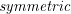### Multiple Degree of Freedom Systems: Influence Coefficients

We have so far used Newton’s laws to obtain the equations of motion for multiple degree of freedom systems. Another way to obtain them is to use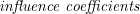. (These are based on Newton’s laws as well, but are often easier to apply.) Influence coefficients relate the effects of forces and displacements on one part of a structure to another and are used extensively in structural engineering. Essentially, one set of influence coefficients can be associated with each matrix appearing in the equations of motion. Just as with the equations of motion, the influence coefficients are directly related to the choice of coordinates in a problem. A different set of coordinates for the same problem will result in a completely different set of influence coefficients.

For the stiffness matrix, there are two (related) sets of influence coefficients that can be used

• Stiffness influence coefficients, and
• Flexibility influence coefficients.

For the mass matrix we can use

• Inertia influence coefficients.

Flexibility Influence Coefficients
The flexibility influence coefficients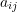are defined as= the deflection of coordinatein the positive direction due to the application of a unit load at coordinate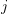in the positive direction.

Note that for a single degree of freedom system this simplifies to our previous definition for the equivalent stiffness. For a multiple degree of freedom system, these coefficients are determined in practice by applying a unit load to the system in the positivecoordinate direction and determining the displacement of all the coordinates in the system. This is a statics problem. Thenis the deflection of the system in the (positive)coordinate direction in this case.

Flexibility influence coefficients can also be applied to torsional systems where= the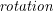at coordinatein the positive direction due to the application of a unit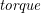at coordinatein the positive direction.

We can also use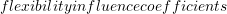in mixed systems with both linear and rotational coordinates. The units ofwill then change depending on whetherandare linear or angular coordinates.
Stiffness Influence Coefficients
The stiffness influence coefficients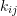are defined as= the force required at coordinatein the positive direction to maintain a unit deflection of coordinatein the positive direction while maintaining zero displacement for all of the other coordinates.

Note that for a single degree of freedom system this simplifies to our previous definition for equivalent stiffness. For a multiple degree of freedom system, these coefficients are determined in practice by considering the system when the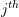coordinate has a positive unit displacement while all other coordinates are held fixed with zero displacement. The forces required to maintain the structure in this deformed configuration are then solved statically.is then the force required in the positivecoordinate direction to maintain this deformed configuration.

Stiffness influence coefficients can also be applied to torsional systems where= therequired at coordinatein the positive direction to maintain a static unitof coordinatein the positive direction while maintaining zero rotation for all of the other coordinates.}

We can also use single degree of freedom in mixed systems with both linear and rotational coordinates. The units ofwill then change depending on whetherandare linear or angular coordinates.
Inertia Influence Coefficients
The inertia influence coefficients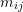are defined as= \text{the impulse that must be applied at coordinatein the positive direction to produce a unit velocity at coordinatein the positive direction while maintaining zero velocity at all the other coordinates.

An alternative equivalent definition is= the force that must be applied at coordinatein the positive direction to produce a unit acceleration at coordinatein the positive direction while maintaining zero acceleration at all the other coordinates.

inertia influence coefficients can also be applied to torsional systems where= theat coordinatein the positive direction that must be applied to produce a unit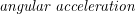at coordinatein the positive direction while maintaining zero angular acceleration at all the other coordinates.

We can also use inertia influence coefficients in mixed systems with both linear and rotational coordinates. The units ofwill then change depending on whetherandare linear or angular coordinates.

### Properties of Stiffness and Flexibility Influence Coefficients

• The total force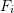acting in the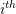coordinate direction can be determined from all of the displacements (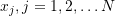) in the system from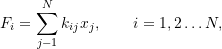or in matrix form

(8.32)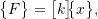where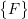is a column vector of the forces acting on the system in the positive coordinate directions and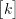is the matrix of single degree of freedom. (Note that if one of the coordinates is an angular displacement, the corresponding term inwould be a torque.)Similarly, the total displacement in thecoordinate direction can be determined from all of the forces acting in the positive coordinate directions (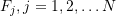) for the system from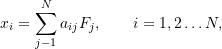or again in matrix form

(8.33)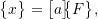where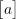is the matrix of flexibility influence coefficients. (Note that if one of the coordinates is an angular displacement, the corresponding term in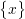would be a rotation.)Substituting (8.33) into (8.32) shows that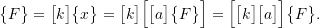Similarly,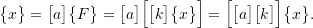These results show that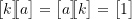which implies that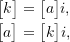or

 The matrices of stiffness and flexibility influence coefficients are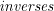of each other.
• For linear systems, Maxwell’s Reciprocity Theorem tells us that the deflection at pointdue to a unit load at pointin a structure is the same as the deflection at pointdue to a unit load at point(all in the positive directions). As a result we find that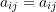which implies that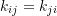. These results lead to the conclusion that
 The matrices of stiffness and flexibility influence coefficients are